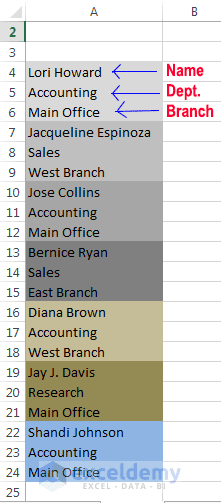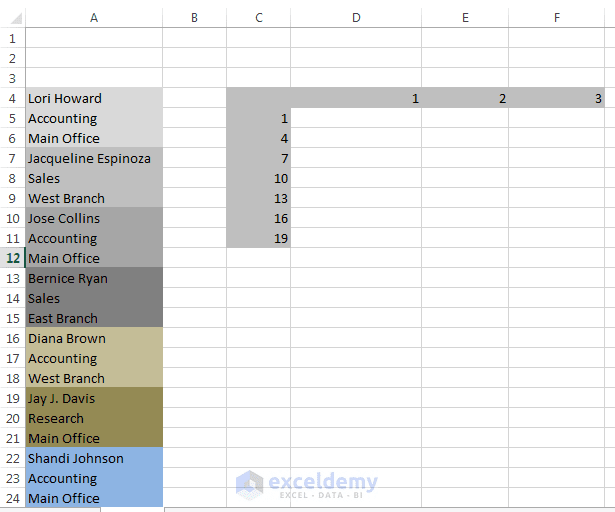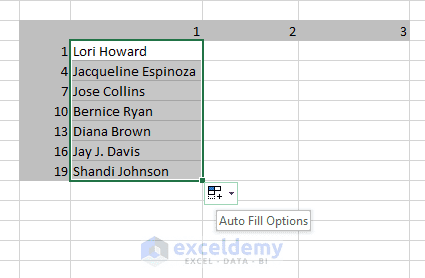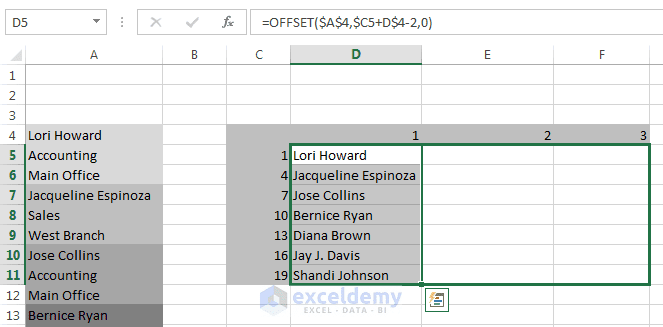Data clean-up techniques in Excel: Changing vertical data to horizontal data

Sometimes after importing a file in your Excel file, the following type of data layout may generate. See the following image.Vertical data. We shall convert this data into three columns. First one shows the name of the employee, second one his/her dept. and the last one is a branch.

Each color-zone in the above image consists of three types of data: Name, Department, and Branch. Our goal is to separate these color-zones in an organized way: names will be under “Name” column, Departments will be under “Department” column and Branches will be under “Branch” column.

There are many ways to convert this type of data, but we are explaining here an easy and simple method. Our method will use a formula, which is copied to a range of cells.

The following steps will narrate the process:

Download this file to work with Changing Vertical Data to horizontal Data.

Step 1:

Create some numeric(1, 2, 3, …) “headers” both vertically and horizontally, as shown in the following figure.Vertical Numeric Headers are 1, 4, 7, 10, 13, 16, 19 & Horizontal Numeric Headers are 1, 2, 3.

Observe our data, you will find that the names are in 1st, 4th, 7th, 10th, 13th, 16th and 19th positions. So we have filled the column C with the values: 1, 4, 7, 10, 13, 16, and 19. You can make a simple formula in cell C6 like this: “=C5+3”. Then copy this formula using fill handle until you reach cell C11. If you don’t know how to do it, click on link how to copy formula in Excel. You can also use Flash Fill technique to fill these cells. Read how to use flash fill in Excel.

The horizontal headers consist of consecutive integers, starting with 1. In our example, color-zone/record contains three cells of data, so the horizontal headers are 1, 2, and 3.

Read More: Data clean-up techniques in Excel: Changing the case of text

Step 2:

Enter the following formula in cell D5: =OFFSET(\$A\$4,\$C5+D\$4-2,0). If you don’t know how to work with OFFSET function click this link: OFFSET Function in Excel.

Copy this formula down to the next six rows. This result is shown in the following figure.Copying formula down to the next six rows.

Now select cell range: D5:D11 and copy this cell range using Fill Handle, this type of copy will actually copy the internal formulas of the cell ranges.Copying formula across to the next 3 columns.

Step 3:

What does this formula work? We shall analyze step by step. We have used here absolute cell reference.

The formulas in column C are like these:

=OFFSET(\$A\$4, \$C5+D\$4-2, 0)=OFFSET(\$A\$4, 0, 0)=\$A\$4= Lori Howard;

This is because: \$C5+D\$4=1+1=2 and \$C5+D\$4-2=2-2=0 and OFFSET(\$A\$4, 0, 0)=\$A\$4.

Note:“\$” sign is used to just say that this cell reference is absolute.

In the same way: =OFFSET(\$A\$4, \$C6+D\$4-2, 0)= OFFSET(\$A\$4, 3, 0)= \$A\$7. Here \$C6=4 and D\$4=1. and \$C6+D\$4-2=4+1-2=3. So OFFSET(\$A\$4, \$C6+D\$4-2, 0)= OFFSET(\$A\$4, 3, 0)= \$A\$7= Jacqueline Espinoza

According to above explanation the following formulas will output these ways:
=OFFSET(\$A\$4, \$C7+D\$4-2, 0)=Jose Collins
=OFFSET(\$A\$4, \$C8+D\$4-2, 0)=Bernice Ryan
=OFFSET(\$A\$4, \$C9+D\$4-2, 0)=Diana Brown
=OFFSET(\$A\$4, \$C10+D\$4-2, 0)=Jay J. Davis
=OFFSET(\$A\$4, \$C11+D\$4-2, 0)=Shandi Johnson

Try yourself: Find out how the formulas in column D and column E works.

You can easily change this technique to work with different type of vertical data. Your vertical data can contain a different number of cells in each color-zone or group. For example, if each color-zone/ record contain ten rows of data, the column C header values would be 1, 11, 21, 31, and so on. The horizontal headers would consist of values 1 through 10 rather than 1 through 3. The formula will be the same.

The formula also uses “mixed” referencing in the second argument (“\$C5+D\$4-2”) of the OFFSET function. The C5 reference has a dollar sign in front of C, so column C is the absolute part of the reference. In the D4 reference, the dollar sign is before the 1, so row 1 is the absolute part of the reference.

Data clean-up techniques in Excel: Fixing trailing minus signs

Data clean-up techniques in Excel: Filling blank cells

Data clean-up techniques in Excel: Matching text in a list

How to Find & Remove Duplicate Rows in Excel

Kawser

Hello! Welcome to my Excel blog! It took me some time to be a fan of Excel. But now I am a die-hard fan of MS Excel. I learn new ways of doing things with Excel and share here. Not only how to guide on Excel, but you will get also topics on Finance, Statistics, Data Analysis, and BI. Stay tuned!

We will be happy to hear your thoughts